As of August 2020 the site you are on (wiki.newae.com) is deprecated, and content is now at rtfm.newae.com.

# Extending AES-128 Attacks to AES-256

(diff) ← Older revision | Latest revision (diff) | Newer revision → (diff)

Many of the tutorials in this wiki discuss attacks on AES-128 encryption. It turns out that its big brother, AES-256, can be attacked by extending the same attacks. This page discusses AES-256 and how to reuse an AES-128 attack to obtain the key.

## The AES-256 Algorithm

In AES-128, we used the following steps to encrypt 16 bytes of plaintext:

1. Use a 16 byte key to generate a key schedule, which is 176 bytes long (11 words made up of 16 bytes).
2. Put the 16 bytes of plaintext into a 4x4 state matrix.
3. Combine the first word of the key schedule with the state.
4. Apply 10 rounds of transformations to the state, involving the key schedule.
5. Retrieve 16 bytes of ciphertext from the state matrix.

The transformations involve several functions which mix together the bytes of the state. These functions are SubBytes(), MixColumns(), and ShiftRows().

AES-256 is not much different from AES-128. The encryption process is:

1. Use a 32 byte key to generate a key schedule, which is 240 bytes long (15 words made up of 16 bytes).
2. Put the 16 bytes of plaintext into a 4x4 state matrix.
3. Combine the first word of the key schedule with the state.
4. Apply 14 rounds of transformations to the state, involving the key schedule.
5. Retrieve 16 bytes of ciphertext from the state matrix.

Notice that most of this algorithm is the same. Earlier, we could attack a target by examining the output of a substitution box; since AES-256 uses these same S-boxes, we should have no problem finding a sensitive point to attack.

The following code is an example of the AES-256 decryption algorithm, written by Ilya O. Levin:

aes_addRoundKey_cpy(buf, ctx->deckey, ctx->key);
aes_shiftRows_inv(buf);
aes_subBytes_inv(buf);

for (i = 14, rcon = 0x80; --i;)
{
if( ( i & 1 ) )
{
aes_expandDecKey(ctx->key, &rcon);
}
aes_mixColumns_inv(buf);
aes_shiftRows_inv(buf);
aes_subBytes_inv(buf);
}


Note that this implementation chooses to expand the key during the decryption process. This order of events isn't a big deal to us - the subBytes() operation will still be visible in a power trace.

## Attacking AES-256 Decryption

From our experience with AES-128, we know that the AES substitution boxes are a good attack point. These boxes are non-linear, so we don't have any problems with nearly-correct key guesses. Since there are S-boxes operating on 1 byte each, we should be able to recover 16 bytes from the SubBytes() function. In the decryption code, this part of the algorithm corresponds to the first three lines:

aes_addRoundKey_cpy(buf, ctx->deckey, ctx->key);
aes_shiftRows_inv(buf);
aes_subBytes_inv(buf);


A diagram of this CPA attack point is:

This was enough for a complete AES-128 attack because there are only 16 bytes of key to look for. However, in AES-256, this only gets us half of the key! We'll need to continue by attacking the next round of the algorithm, which consists of one iteration through the following loop:

for (i = 14, rcon = 0x80; --i;)
{
if( ( i & 1 ) )
{
aes_expandDecKey(ctx->key, &rcon);
}
aes_mixColumns_inv(buf);
aes_shiftRows_inv(buf);
aes_subBytes_inv(buf);
// <--- Attack the contents of buf at this point
}


A diagram of this second attack point is:

The critical difference between the initial round and this round is the addition of the mixColumns() operation. This operation takes four bytes of input and generates four bytes of output - any change in a single byte will result in a change of all four bytes of output! It would at first appear that we need to perform a guess over 4 bytes instead of 1 byte. This would be a considerably more complicated operation! To solve this, we can consider writing that last step as an equation. The state at the end of round 13 is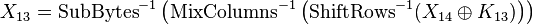$X_{13} = \text{SubBytes}^{-1}\left(\text{MixColumns}^{-1}\left(\text{ShiftRows}^{-1}(X_{14} \oplus K_{13})\right)\right)$

where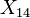$X_{14}$ is the output of round 14,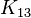$K_{13}$ is the 16 byte round key for round 13, and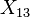$X_{13}$ is the output of round 13 (our attack point). We can simplify this by noticing that MixColumns() is a linear function; that is,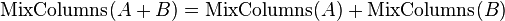$\text{MixColumns}(A + B) = \text{MixColumns}(A) + \text{MixColumns}(B)$

Using this property, we can write down the hypothetical key for round 13, which is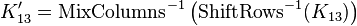$K'_{13} = \text{MixColumns}^{-1}\left(\text{ShiftRows}^{-1}(K_{13})\right)$

and we can use this hypothetical key to calculate the output as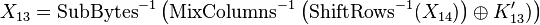$X_{13} = \text{SubBytes}^{-1}\left(\text{MixColumns}^{-1}\left(\text{ShiftRows}^{-1}(X_{14})\right) \oplus K'_{13})\right)$

Using this hypothetical key, we can perform a regular attack to recover each subkey one byte at a time. Then, we can recover the actual round key by calculating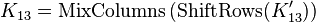$K_{13} = \text{MixColumns}\left(\text{ShiftRows}(K'_{13})\right)$

Using these new equations, we can perform a full attack on AES-256 with the following steps:

1. Perform a regular attack on the first S-box output to recover all 16 bytes of the 14th round key.
2. Using the known values of the 14th round key, calculate the outputs of the second S-box. Use this attack point to recover all 16 bytes of the hypothetical 13th round key.
3. Transform the hypothetical round key into the actual value of the 13th round key.
4. Reverse the 13th and 14th round keys using the AES-256 key schedule to determine the 256 bit secret encryption key.

Once this is done, we have successfully attacked an AES-256 implementation by looking at two separate AES S-boxes.